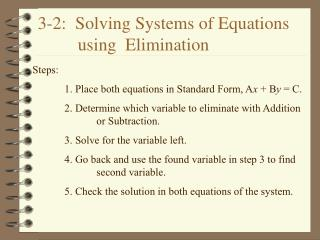DownloadDownload Presentation3-2: Solving Systems of Equations using Elimination

# 3-2: Solving Systems of Equations using Elimination

Download Presentation## 3-2: Solving Systems of Equations using Elimination

- - - - - - - - - - - - - - - - - - - - - - - - - - - E N D - - - - - - - - - - - - - - - - - - - - - - - - - - -
##### Presentation Transcript

1. 3-2: Solving Systems of Equations using Elimination Steps: 1. Place both equations in Standard Form, Ax + By = C. 2. Determine which variable to eliminate with Addition or Subtraction. 3. Solve for the variable left. 4. Go back and use the found variable in step 3 to find second variable. 5. Check the solution in both equations of the system.

2. 5x + 3y = 11 5x = 2y + 1 EXAMPLE #1: STEP1: Write both equations in Ax + By = C form. 5x + 3y =1 5x - 2y =1 STEP 2: Use subtraction to eliminate 5x. 5x + 3y =11 5x + 3y = 11 -(5x - 2y =1) -5x + 2y = -1 Note: the (-) is distributed. STEP 3:Solve for the variable. 5x + 3y =11 -5x + 2y = -1 5y =10 y = 2

3. 5x + 3y = 11 5x = 2y + 1 STEP 4: Solve for the other variable by substituting into either equation. 5x + 3y =11 5x + 3(2) =11 5x + 6 =11 5x = 5 x = 1 The solution to the system is (1,2).

4. 5x + 3y= 11 5x = 2y + 1 Step 5:Check the solution in both equations. The solution to the system is (1,2). 5x = 2y + 1 5(1) = 2(2) + 1 5 = 4 + 1 5=5 5x + 3y = 11 5(1) + 3(2) =11 5 + 6 =11 11=11

5. Solving Systems of Equations using Elimination Steps: 1. Place both equations in Standard Form, Ax + By = C. 2. Determine which variable to eliminate with Addition or Subtraction. 3. Solve for the remaining variable. 4. Go back and use the variable found in step 3 to find the second variable. 5. Check the solution in both equations of the system.

6. Example #2: x + y = 10 5x – y = 2 Step 1: The equations are already in standard form: x + y = 10 5x – y = 2 Step 2: Adding the equations will eliminate y. x + y = 10 x + y = 10 +(5x – y = 2) +5x – y = +2 Step 3:Solve for the variable. x + y = 10 +5x – y = +2 6x = 12 x = 2

7. x + y = 10 5x – y = 2 Step 4: Solve for the other variable by substituting into either equation. x + y = 10 2 + y = 10 y = 8 Solution to the system is (2,8).

8. x + y = 10 5x – y = 2 Step 5:Check the solution in both equations. Solution to the system is (2,8). x + y =10 2 + 8 =10 10=10 5x – y =2 5(2) - (8) =2 10 – 8 =2 2=2

9. NOW solve these using elimination: 1. 2. 2x + 4y =1 x - 4y =5 2x – y =6 x + y = 3

10. Using Elimination to Solve a Word Problem: Two angles are supplementary. The measure of one angle is 10 degrees more than three times the other. Find the measure of each angle.

11. Using Elimination to Solve a Word Problem: Two angles are supplementary. The measure of one angle is 10 more than three times the other. Find the measure of each angle. x = degree measure of angle #1 y = degree measure of angle #2 Therefore x + y = 180

12. Using Elimination to Solve a Word Problem: Two angles are supplementary. The measure of one angle is 10 more than three times the other. Find the measure of each angle. x + y = 180 x =10 + 3y

13. Using Elimination to Solve a Word Problem: Solve x + y = 180 x =10 + 3y x + 42.5 = 180 x = 180 - 42.5 x = 137.5 (137.5, 42.5) x + y = 180 -(x - 3y = 10) 4y =170 y = 42.5

14. Using Elimination to Solve a Word Problem: The sum of two numbers is 70 and their difference is 24. Find the two numbers.

15. Using Elimination to Solve a Word problem: The sum of two numbers is 70 and their difference is 24. Find the two numbers. x = first number y = second number Therefore, x + y = 70

16. Using Elimination to Solve a Word Problem: The sum of two numbers is 70 and their difference is 24. Find the two numbers. x + y = 70 x – y = 24

17. Using Elimination to Solve a Word Problem: x + y =70 x - y = 24 47 + y = 70 y = 70 – 47 y = 23 2x = 94 x = 47 (47, 23)

18. Now you Try to Solve These Problems Using Elimination. Solve • Find two numbers whose sum is 18 and whose difference is 22. • The sum of two numbers is 128 and their difference is 114. Find the numbers.